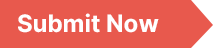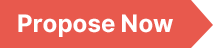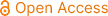### Applied and Computational Mathematics

Archive
• 2023, Volume 12

• 2022, Volume 11

• 2021, Volume 10

• 2020, Volume 9

• 2019, Volume 8

• 2018, Volume 7

• 2017, Volume 6

• 2016, Volume 5

• 2015, Volume 4

• 2014, Volume 3

• 2013, Volume 2

• 2012, Volume 1

Submit a Manuscript

Publishing with us to make your research visible to the widest possible audience.Propose a Special Issue

Building a community of authors and readers to discuss the latest research and develop new ideas.### Lagrange-type Algebraic Minimal Bivariate Fractal Interpolation Formula

Fractal interpolation methods became an important method in data processing, even for functions with abrupt changes. In the last few decades it has attracted several authors because it can be applied in various fields. The advantage of these methods are that we can generalize the classical approximation methods and also we can combine these methods for example with Lagrange interpolation, Hermite interpolation or spline interpolation. The classical Lagrange interpolation problem give the construction of a suitable approximate function based on the values of the function on given points. These method was generalized for more than one variable functions. In this article we generalize the so-called algebraic maximal Lagrange interpolation formula in order to approximate functions on a rectangular domain with fractal functions. The construction of the fractal function is made with a so-called iterated function system. This method it has the advantage that all classical methods can be obtained as a particular case of a fractal function. We also use the construction for a polynomial type fractal function and we proof that the Lagrange-type algebraic minimal bivariate fractal function satisfies the required interpolation conditions. Also we give a delimitation of the error, using the result regarding the error of a polynomial fractal interpolation function.

Fractal Interpolation, Lagrange Interpolation, Fractal Surfaces

Ildikó Somogyi, Anna Soós. (2023). Lagrange-type Algebraic Minimal Bivariate Fractal Interpolation Formula. Applied and Computational Mathematics, 12(5), 109-113. https://doi.org/10.11648/j.acm.20231205.11